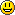#创客百科

### 侧边栏

• 树莓派瑞士军刀扩展板

Arduino

MicroPython

micro:bit

en:arduino:language:bitwisecompoundand

# compound bitwise AND (&=)

## Description

The compound bitwise AND operator (&=) is often used with a variable and a constant to force particular bits in a variable to the LOW state (to 0). This is often referred to in programming guides as "clearing" or "resetting" bits.

## Syntax:

`x &= y;   // equivalent to x = x & y; `

## Parameters

x: a char, int or long variable

y: an integer constant or char, int, or long

## Example:

First, a review of the Bitwise AND (&) operator

```   0  0  1  1    operand1
0  1  0  1    operand2
----------
0  0  0  1    (operand1 & operand2) - returned result```

Bits that are “bitwise ANDed” with 0 are cleared to 0 so, if myByte is a byte variable,

`myByte & B00000000 = 0;`

Bits that are “bitwise ANDed” with 1 are unchanged so,

`myByte & B11111111 = myByte;`

Note: because we are dealing with bits in a bitwise operator - it is convenient to use the binary formatter with constants. The numbers are still the same value in other representations, they are just not as easy to understand. Also, B00000000 is shown for clarity, but zero in any number format is zero (hmmm something philosophical there?) Consequently - to clear (set to zero) bits 0 & 1 of a variable, while leaving the rest of the variable unchanged, use the compound bitwise AND operator (&with the constant B11111100

```   1  0  1  0  1  0  1  0    variable
1  1  1  1  1  1  0  0    mask
----------------------
1  0  1  0  1  0  0  0

variable unchanged
bits cleared
```

Here is the same representation with the variable's bits replaced with the symbol x

```   x  x  x  x  x  x  x  x    variable
1  1  1  1  1  1  0  0    mask
----------------------
x  x  x  x  x  x  0  0

variable unchanged
bits cleared
```

So if:

```myByte =  10101010;

myByte &= B1111100 == B10101000;```

en/arduino/language/bitwisecompoundand.txt · 最后更改: 2016/12/25 22:15 (外部编辑)

### 页面工具